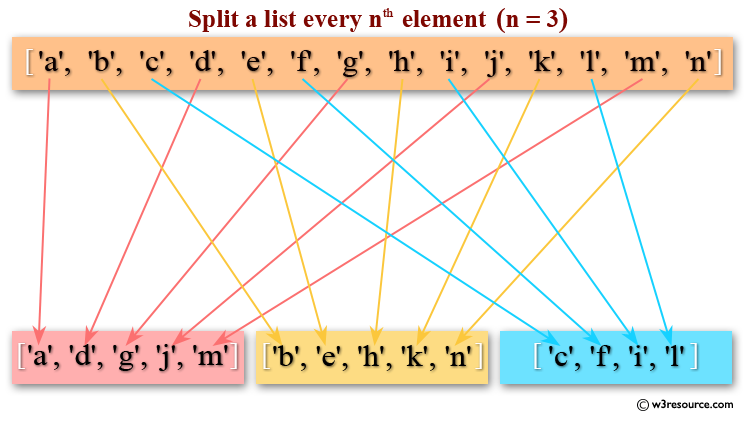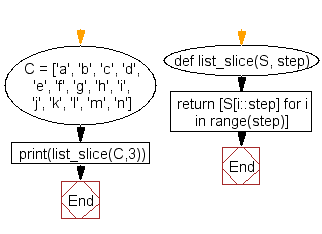﻿ Python: Split a list every Nth element - w3resource

# Python: Split a list every Nth element

## Python List: Exercise - 51 with Solution

Write a Python program to split a list every Nth element.Sample Solution:-

Python Code:

``````C = ['a', 'b', 'c', 'd', 'e', 'f', 'g', 'h', 'i', 'j', 'k', 'l', 'm', 'n']
def list_slice(S, step):
return [S[i::step] for i in range(step)]
print(list_slice(C,3))
```
```

Sample Output:

```[['a', 'd', 'g', 'j', 'm'], ['b', 'e', 'h', 'k', 'n'], ['c', 'f', 'i', 'l']]
```

Flowchart:## Visualize Python code execution:

The following tool visualize what the computer is doing step-by-step as it executes the said program:

Python Code Editor:

Have another way to solve this solution? Contribute your code (and comments) through Disqus.

What is the difficulty level of this exercise?

Test your Programming skills with w3resource's quiz.

﻿

## Python: Tips of the Day

Decapitalizes the first letter of a string:

Example:

```def tips_decapitalize(s, upper_rest=False):
return s[:1].lower() + (s[1:].upper() if upper_rest else s[1:])
print(tips_decapitalize('PythonTips'))
print(tips_decapitalize('PythonTips', True))
```

Output:

```pythonTips
pYTHONTIPS
```

We are closing our Disqus commenting system for some maintenanace issues. You may write to us at reach[at]yahoo[dot]com or visit us at Facebook